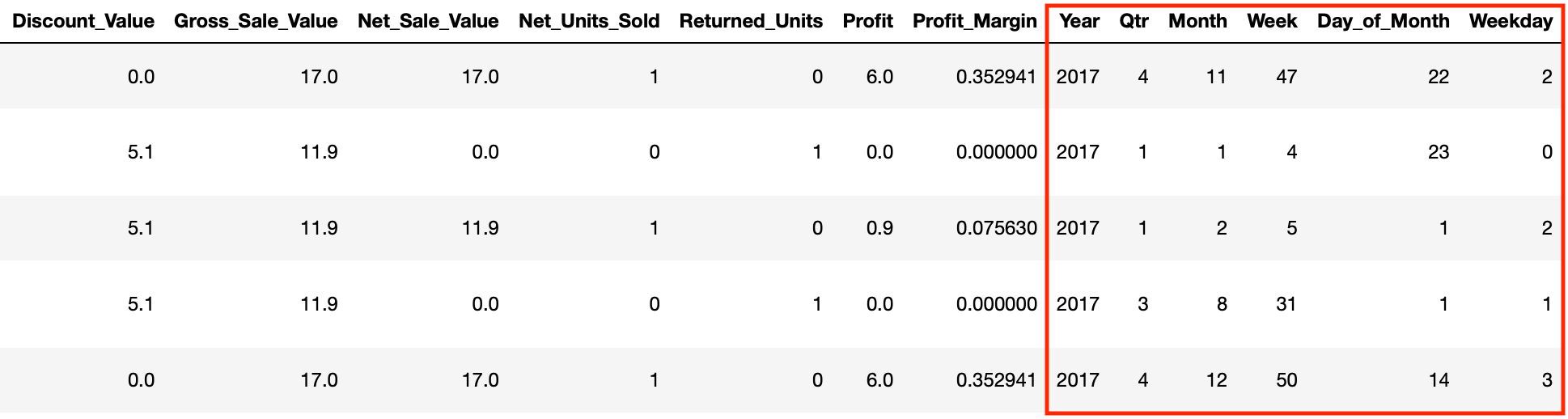# Pandas Dates

Pandas comes with inbuilt operations to extract date and time features from date time columns and these can in turn be used to create new columns. We do this by using the dt object and then specifying the date feature we want to extract from our date column i.e. year or month.

df[date_column].dt.year

In our data set we know the date that an order was placed so let’s create some new columns containing different date features that we can use for reporting and modelling purposes later on. We are going to the get the year, quarter, month, week, day of month and weekday that each order occurred.

df['Year'] = df['Order_Date'].dt.year
df['Qtr'] = df['Order_Date'].dt.quarter
df['Month'] = df['Order_Date'].dt.month
df['Week'] = df['Order_Date'].dt.week
df['Day_of_Month'] = df['Order_Date'].dt.day
df['Weekday'] = df['Order_Date'].dt.weekdayNext